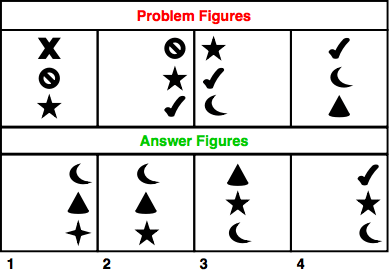# Non-Verbal Analogies - Logical Reasoning (MCQ) Questions for Q. 30060

Q.  The following question consists of some Problem Figures followed by other figures marked 1, 2, 3, 4 called the Answer Figures.

Find out the correct answer figure that should come next in the sequence of problem figures.- Published on 15 May 17

a. 1
b. 2
c. 3
d. 4

2nd element becomes the top element in the next figure. Third element becomes second element. The previous element is deleted and replaced with another.

The complete column of figures moves one-third step forward each time. Thus, the answer figure should be figure 1.

#### Discussion

• Bhavik    -Posted on 04 Apr 20
Need more practice questions

## ➨ Post your comment / Share knowledgeEnter the code shown above:

(Note: If you cannot read the numbers in the above image, reload the page to generate a new one.)